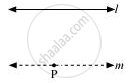# How Would You Rewrite Euclid’S Fifth Postulate So that It Would Be Easier to Understand? - Mathematics

How would you rewrite Euclid’s fifth postulate so that it would be easier to understand?

#### Solution

Two lines are said to be parallel if they are equidistant from one other and they do not have any point of intersection. In order to understand it easily, let us take any line and a point P not on l. Then, by Playfair’s axiom (equivalent to the fifth postulate), there is a unique line m through P which is parallel to l.The distance of a point from a line is the length of the perpendicular from the point to the line. Let AB be the distance of any point on m from l and CD be the distance of any point on l from m. It can be observed that AB = CD. In this way, the distance will be the same for any point on m from l and any point on l from m. Therefore, these two lines are everywhere equidistant from one another.Concept: Equivalent Versions of Euclid’S Fifth Postulate
Is there an error in this question or solution?
Chapter 5: Introduction to Euclid's Geometry - Exercise 5.2 [Page 88]

#### APPEARS IN

NCERT Class 9 Maths
Chapter 5 Introduction to Euclid's Geometry
Exercise 5.2 | Q 1 | Page 88

Share